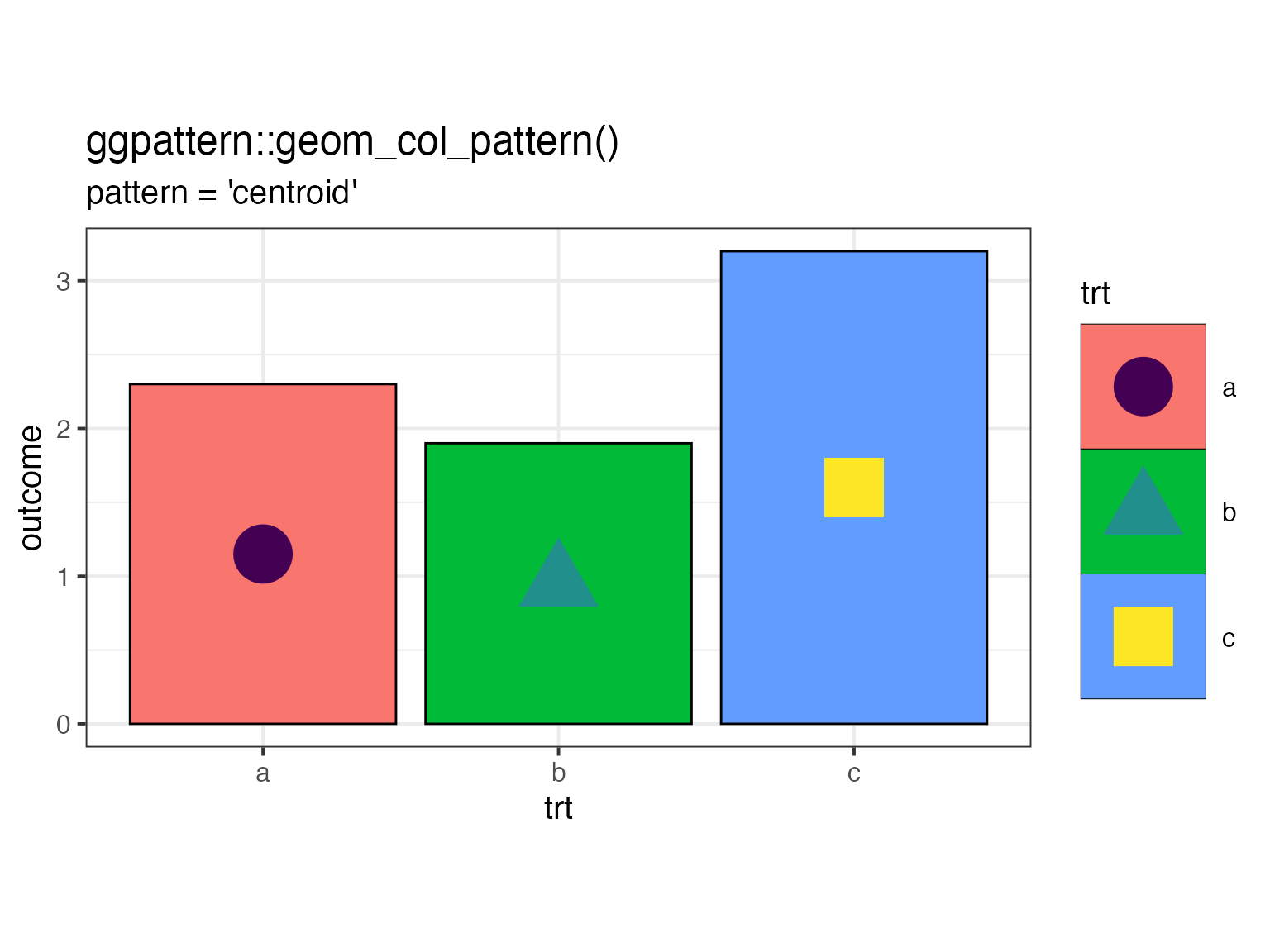library(ggplot2)
library(ggpattern)

## Introduction

This vignette shows how to:

• write a create_pattern() function of the correct signature for a geometry-based pattern
• Instruct ggpattern on where to find this user defined pattern

## Write the geometry-based pattern function

All geometry-based pattern creation functions must:

1. Have the exact function signature: function(params, boundary_df, aspect_ratio, legend)
• params - parameters from the geom (the aesthetics) e.g pattern_fill
• boundary_df - data.frame containing polygon information i.e. The polygon_df format.
• aspect_ratio - the best guess that ggpattern is able to make as to the aspect ratio of the viewport in which this pattern is being rendered.
• legend logical value to indicate whether or not this function is being called to render a key legend or the in-place geom fill.
2. Return a grid grob object. This can be any valid grob including a grid::grobTree()

Parameters for this pattern:

• pattern_shape to indicate the character to use for plotting
• pattern_size size of the plotted character
• pattern_fill colour of the character
• pattern_alpha character transparence
#~~~~~~~~~~~~~~~~~~~~~~~~~~~~~~~~~~~~~~~~~~~~~~~~~~~~~~~~~~~~~~~~~~~~~~~~~~~~~
#' Create a single character pointsGrob at the cenroid of the geom area
#'
#' @param params params/coords for a single element. named list or single row data.frame
#' @param boundary_df mask for the pattern rendering
#' @param aspect_ratio a aspect ratio of the plotting area.
#' @param legend is the pattern being created in the legend? default FALSE.
#'  Use this flag if you want different pattern drawing behaviour for the legend.
#'
#' @return grid grob object
#~~~~~~~~~~~~~~~~~~~~~~~~~~~~~~~~~~~~~~~~~~~~~~~~~~~~~~~~~~~~~~~~~~~~~~~~~~~~~
centroid_dot_pattern <- function(params, boundary_df, aspect_ratio, legend) {

#~~~~~~~~~~~~~~~~~~~~~~~~~~~~~~~~~~~~~~~~~~~~~~~~~~~~~~~~~~~~~~~~~~~~~~~~~~~
# Convert the simple boundary_df polygon information into a
# simple features polygon object i.e. {sf}
#~~~~~~~~~~~~~~~~~~~~~~~~~~~~~~~~~~~~~~~~~~~~~~~~~~~~~~~~~~~~~~~~~~~~~~~~~~~
boundary_sf <- ggpattern::convert_polygon_df_to_polygon_sf(boundary_df)

#~~~~~~~~~~~~~~~~~~~~~~~~~~~~~~~~~~~~~~~~~~~~~~~~~~~~~~~~~~~~~~~~~~~~~~~~~~~
# Now that we have the boundary as an {sf} object, we can use a simple
# features' function to find the centroid
#~~~~~~~~~~~~~~~~~~~~~~~~~~~~~~~~~~~~~~~~~~~~~~~~~~~~~~~~~~~~~~~~~~~~~~~~~~~
centroid    <- sf::st_centroid(boundary_sf)

#~~~~~~~~~~~~~~~~~~~~~~~~~~~~~~~~~~~~~~~~~~~~~~~~~~~~~~~~~~~~~~~~~~~~~~~~~~~
# Create a single character at the cenroid
#~~~~~~~~~~~~~~~~~~~~~~~~~~~~~~~~~~~~~~~~~~~~~~~~~~~~~~~~~~~~~~~~~~~~~~~~~~~
grid::pointsGrob(
x    = centroid,
y    = centroid,
pch  = params$pattern_shape, size = unit(params$pattern_size, 'char'),
gp   = grid::gpar(
col = ggplot2::alpha(params$pattern_fill, params$pattern_alpha)
)
)
}

## Let {ggpattern} know that there’s an external pattern function it can use

A global option (ggpattern_geometry_funcs) is a named list which contains geometry-based pattern creating functions to use outside of ggpattern.

The name used in this list corresponds to the pattern name used with the geom - in this case we will be using pattern = 'centroid'.

options(ggpattern_geometry_funcs = list(centroid = centroid_dot_pattern))

## Use this centroid pattern

There is an included pattern = 'none' in ggpattern.

Here, a verbose version of the ‘none’ pattern is created. It still just returns an empty grob, but as a side-effect prints out the parameters set for this object.

df <- data.frame(
trt     = c("a", "b", "c"),
outcome = c(2.3, 1.9, 3.2)
)

ggplot(df, aes(trt, outcome)) +
geom_col_pattern(
aes(
fill          = trt,
pattern_shape = trt,
pattern_fill  = trt
),
colour          = 'black',
pattern         = 'centroid',
pattern_size    = 3
) +
theme_bw(15) +
labs(
title    = "ggpattern::geom_col_pattern()",
subtitle = "pattern = 'centroid'"
) +
scale_pattern_fill_viridis_d() +
theme(legend.key.size = unit(2, 'cm')) +
coord_fixed(ratio = 1/2)
#> Loading required namespace: sf